# "Proximity" game where masses are taken or taken out by the size of numbers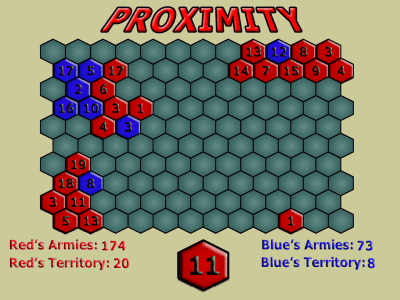You can put a randomly chosen number in the square, you can increase the number by 1 when you are next to your square, and when you are next to an enemy's square you can catch that mass if the number is bigger game. Rules are hard to understand if it is sentences, but it is a simple game that you can understand as soon as you play.

Access is from the following.
Newgrounds presents: Proximity

title. The rules are written.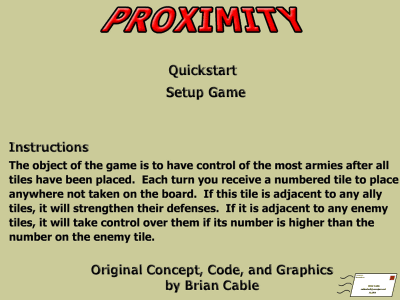In the initial setting, the player is in charge of red, the computer is in charge of blue. "Armies" is the total number, "Territoty" is the number of squares controlling.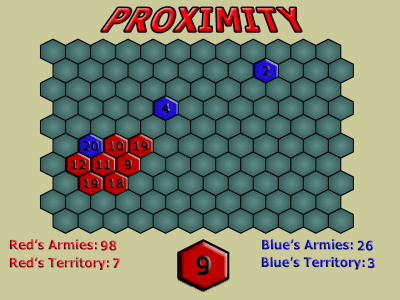Red is considerably leading if it is the sum of figures, but the number of troops is close battle.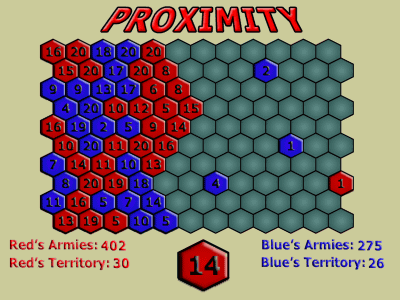Red turn, number is "19". The figure is quite strong because it is 20 at the maximum. When it is placed in a yellow place, 12 and 15 of blue become a red mass.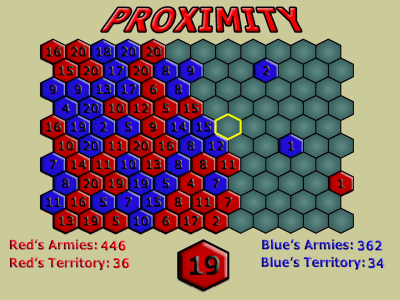Even at the end, the number of trousers also leads considerably. However, since the number to put is random irrespective of the number of squares taken so far, it may be reversed in the second half.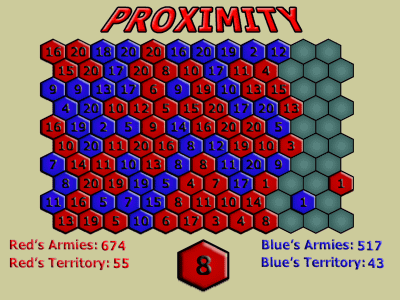At the end I was pretty stuck, but victory.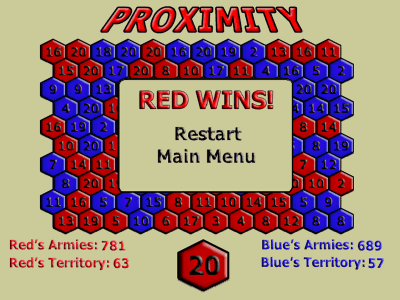in Review,   Game, Posted by logc_nt